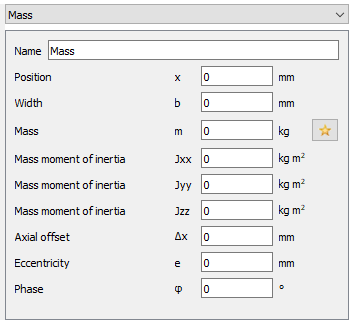﻿ Mass

# MESYS Calculation Software

 MassAn additional mass can be defined using the “Mass” element. In addition to its mass, also the mass moment of inertia around three axes can be defined. The weight of the mass is considered and it is considered in the calculation of natural frequencies. The-button allows to calculate mass and inertia based on a cylinder geometry.

If a width is entered, the mass is equally distributed on this line. The total mass inertia of this distributed mass are the input values, which results in a minimum value for Jyy and Jzz. A message will be shown if the input values are too small. The axial offset is the axial distance between center of width of the load element and the center of mass.

If the added mass has an eccentricity, it will cause rotating unbalance and can be considered as a harmonic force according to the formula: (ecc: eccentricity in mm). A phase shift, ϕ (°), for the imbalance force can be taken into account too. The imbalance will only affect the results for the harmonic response. Bearing life, contact stress or safety factors for shaft strength will not change. Setting the option Consider dynamic loads in static equlibrium will lead to a change of bearing life or safety factors, but this is an approximation only.